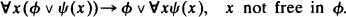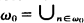Home

# Craig interpolation theorem for intuitionistic logic and extensions Part III

## Extract

This is a continuation of two previous papers by the same title  and examines mainly the interpolation property for the logic CD with constant domains, i.e., the extension of the intuitionistic predicate logic with the schemaIt is known ,  that this logic is complete for the class of all Kripke structures with constant domains.

Theorem 47. The strong Robinson consistency theorem is not true for CD.

Proof. Consider the following Kripke structure with constant domains. The set S of possible worlds is ω0, the set of positive integers. R is the natural ordering ≤. Let ω0 0 =, Bn, is a sequence of pairwise disjoint infinite sets. Let L0 be a language with the unary predicates P, P1 and consider the following extensions for P,P1 at the world m.

(a) P is true on ⋃i≤2nBi, and P1 is true on ⋃i≤2n+1Bi for m = 2n.

(b) P is true on ⋃i≤2nBi, and P1 for ⋃i≤2n+1Bi for m = 2n.

Let (Δ,Θ) be the complete theory of this structure. Consider another unary predicate Q. Let L be the language with P, Q and let M be the language with P1, Q.

Hide All

# Craig interpolation theorem for intuitionistic logic and extensions Part III

## Metrics

### Full text viewsFull text views reflects the number of PDF downloads, PDFs sent to Google Drive, Dropbox and Kindle and HTML full text views.

Total number of HTML views: 0
Total number of PDF views: 0 *Loading metrics...

### Abstract viewsAbstract views reflect the number of visits to the article landing page.

Total abstract views: 0 *Loading metrics...

* Views captured on Cambridge Core between <date>. This data will be updated every 24 hours.

Usage data cannot currently be displayed.